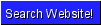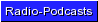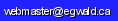Egwald Statistics: Restricted Multiple Regression

by

Elmer G. Wiens

Egwald's popular web pages are provided without cost to users.
Please show your support by joining Egwald Web Services as a Facebook Fan:Follow Elmer Wiens on Twitter:Cobb-Douglas Production Function

I assume you understand Part A. Multiple Regression Analysis. I will extend the model so you can place linear restrictions on the parameters that you want to estimate from a set of data.

For estimates of the coefficients of other production functions see:

Consider the three factor Cobb-Douglas Production Function:

Y = A * (L^alpha) * (K^beta) * (M^gamma)

where L = labour, K = capital, and M = materials and supplies.

You have a set of data with 15 observations. You want to estimate the parameters alpha, beta, and gamma. Taking the logarithm of both sides of the equation, and renaming the parameters:

logY = ß0 + ß1*logL + ß2*logK + ß3*logM

gives us a linear equation. I want to place some restrictions on the parameters.

.92 = ß1 + ß2 + ß3
.33 = ß0 + ß1

I have 15 observations, 3 independent variables (don't include the constant term A), and 2 linear restrictions on the parameters to be estimated. I want an intercept ß0 = logA.

To keep things manageable, the number of observations must be less than 20, the number of independent variables less than 6, and the number of linear restrictions must be less than 5. The number of observations must be greater than or equal to the number of variables.

Regression title
#obser
vations
#indep
vars
#linear
restricts
Intercept
term
Yes No
Give your study a name and enter it with the number of observations, independent variables, and linear restrictions into the table. Then click 'submit parameters'.Copyright © Elmer G. Wiens:   Egwald Web ServicesAll Rights Reserved.    Inquiries